# KSEEB SSLC Class 10 Maths Solutions Chapter 11 Introduction to Trigonometry Ex 11.4

In this chapter, we provide KSEEB SSLC Class 10 Maths Solutions Solutions Chapter 11 Introduction to Trigonometry Ex 11.4 for English medium students, Which will very helpful for every student in their exams. Students can download the latest KSEEB SSLC Class 10 Maths Solutions Solutions Chapter 11 Introduction to Trigonometry Ex 11.4 pdf, free KSEEB SSLC Class 10 Maths Solutions Solutions Chapter 11 Introduction to Trigonometry Ex 11.4 pdf download. Now you will get step by step solution to each question.

### Karnataka State Syllabus Class 10 Maths SolutionsChapter 11 Introduction to Trigonometry Ex 11.4

Question 1.
Express the trigonometric ratios sin A, sec A and tan A in terms of cot A.
Solution:
cosec2 A – cot2 A = 1
cosec2 A = 1 + cot2 A
cosec2 A = cot2 A + 1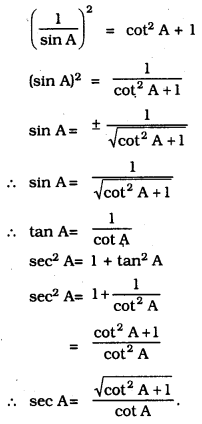Question 2.
Write all the other trigonometric ratios of ∠A in terms of sec A.
Solution:
i) sin2 A + cos2 A = 1
sin2 A = 1 – cos2 A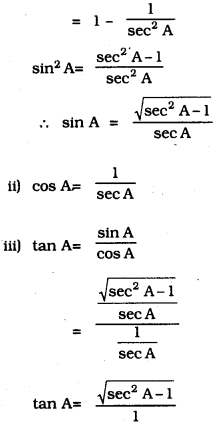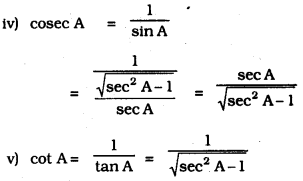Question 3.
Evaluate :
i) sin263∘+sin27∘cos217∘+cos273∘
ii) sin 25° cos 65° + cos 25° sin 65°
Solution: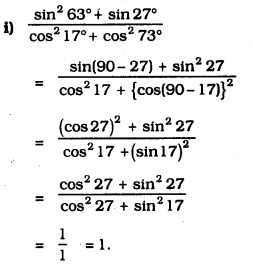ii) sin 25° cos 65° + cos 25° sin 65°
= sin 25° cos (90° – 25°) + cos 25° sin (90° – 25°)
= sin 25° sin 25° + cos 25° + cos 25°
= sin2 25° + cos2 25°
= 1. [∵ cos2 θ + sin2 θ = 1]

Question 4.
Choose the correct option. Justify your choice.
i) 9 sec2 A – 9 tan2 A.
A) 1
B) 9
C) 8
D) 0
Solution:
B) 9
9 sec2 A – 9 tan2 A
= 9(sec2 A – tan2 A)
= 9 × 1
= 9

ii) (1+tan θ + sec θ) (1+ cot θ- cosec θ) =
A) 0
B) 1
C) 2
D) -1
Solution:
C) 2
(1+tan θ + sec θ) (1+ cot θ- cosec θ)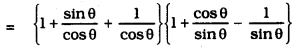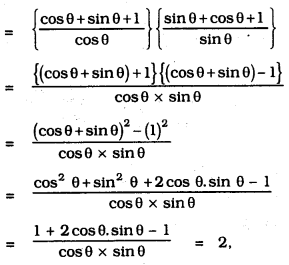iii) (sec A + tan A) (1 – sin A) =
A) sec A
B) sin A
C) cosec A
D) cos A
Solution:
D) cos A
(sec A + tan A) (1 – sin A)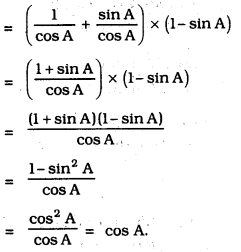iv) 1+tan2A1+cot2A =
A) sec2 A
B) -1
C) cot2 A
D) tan2 A
Solution:
D) tan2 A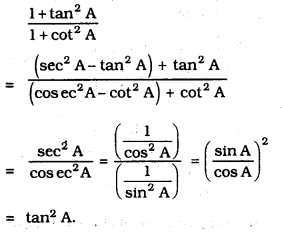Question 5.
Prove the following identities, where the angles involved are acute angles for which the expressions are defined.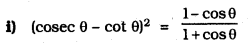Solution: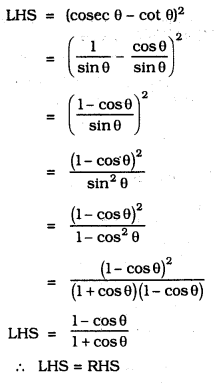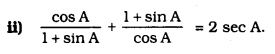Solution: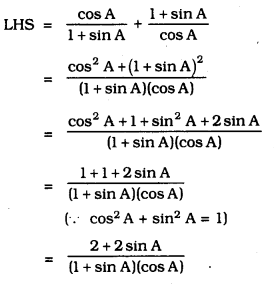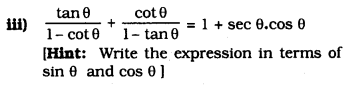Solution: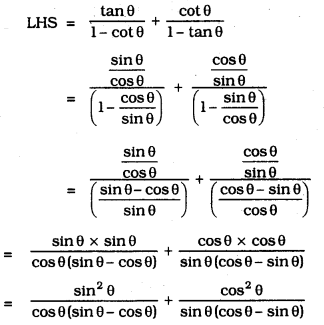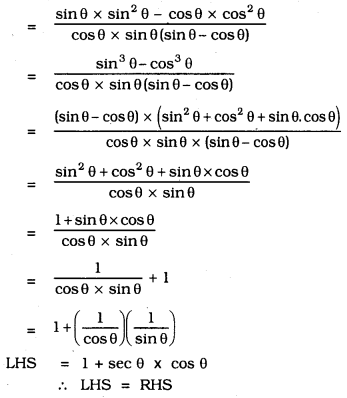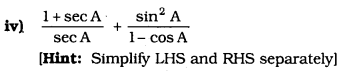Solution: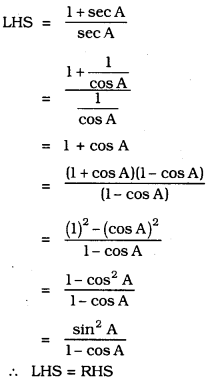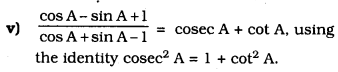Solution: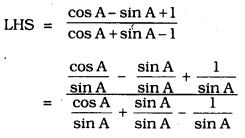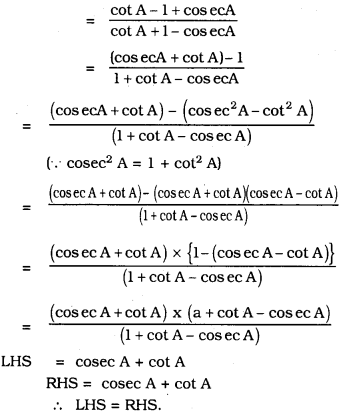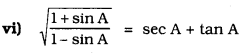Solution: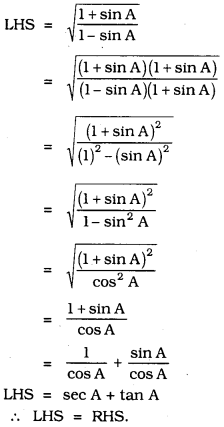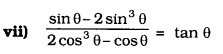Solution: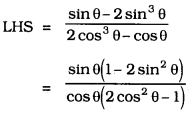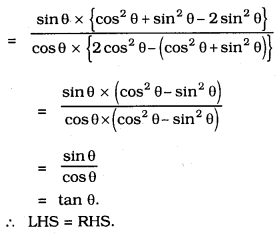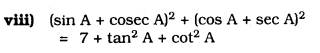Solution: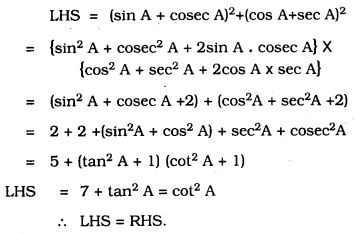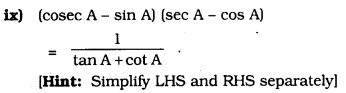Solution: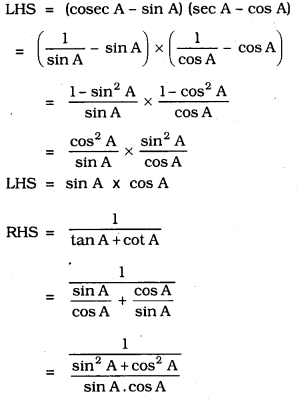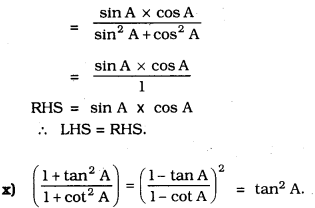Solution: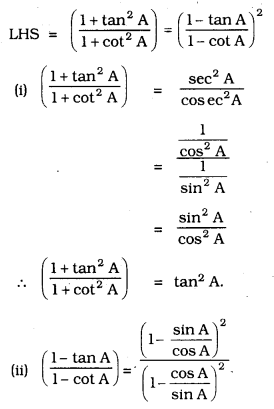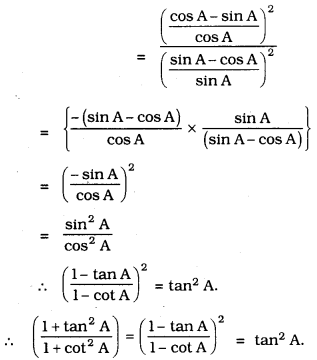All Chapter KSEEB Solutions For Class 10 Maths

—————————————————————————–

All Subject KSEEB Solutions For Class 10

*************************************************

I think you got complete solutions for this chapter. If You have any queries regarding this chapter, please comment on the below section our subject teacher will answer you. We tried our best to give complete solutions so you got good marks in your exam.

If these solutions have helped you, you can also share kseebsolutionsfor.com to your friends.

Best of Luck!!